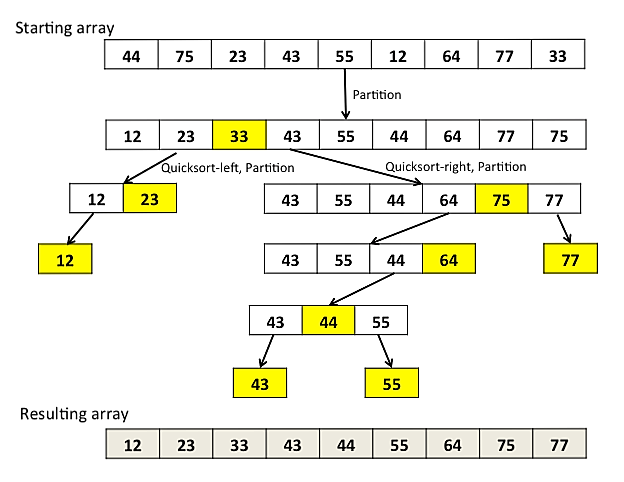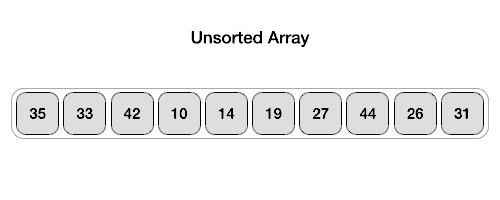# Quicksort algorithm implementation in java programming

As the name, quicksort is the quickest algorithm to sort some numbers. There are some other sorting algorithms like bucket sort, insertion sort, bubble sort, radix sort, etc. Among them, quicksort is very efficient. The efficacy of quicksort in the worst cause is(n2), in the best cause(nLogn), and in the average cause, O (N log N).

Here is the step diagram of quicksort:Quicksort algorithm

Here is the animated simulation of a quick sort algorithm.Quicksort algorithm implementation

If we look closely, the quick sort algorithm selects the last position of the array and assumes it as a pivot. It took two variables and assign the most left and the rightest value of the array. Then it compares them with the pivot and swaps if the left number is smaller than then the right number.

The algorithm steps will explain the quick sort easily:

Step 1 − First, Choose the highest index value of array has pivot
Step 2 − Take two variables and assign the most left and the rightest value of the array excluding pivot
Step 3 − left variable points to the low index of the array
Step 4 − right variable points to the high index of the array
Step 5 − if value at left variable is less than pivot, move right
Step 6 − if value at right variable is greater than pivot move left
Step 7 − if both step 5 and step 6 does not match swap left and right
Step 8 − if left ≥ right, the point where they met is new pivot.

Let's look at the Quick sort algorithm implementation in java code:

Happy Coding.